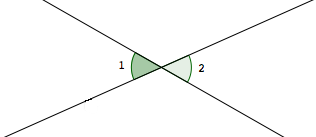﻿ Angles: Complementary, Supplementary, Vertical, and Linear Pairs
ANGLES: COMPLEMENTARY, SUPPLEMENTARY,
VERTICAL, and LINEAR PAIRS
by Dr. Carol JVF Burns (website creator)
Follow along with the highlighted text while you listen!

For this web exercise, assume all angles are measured in degrees.

DEFINITIONS complementary angles, supplementary angles
Two angles are complementary   iff   the sum of their measures is $\,90^{\circ}\,$.

Two angles are supplementary   iff   the sum of their measures is $\,180^{\circ}\,$.

In particular:

• if $\,\angle 1\,$ and $\,\angle 2\,$ are complementary, then $\,m\angle 1+ m\angle 2 = 90^{\circ}\,$
• if $\,\angle 1\,$ and $\,\angle 2\,$ are supplementary, then $\,m\angle 1+ m\angle 2 = 180^{\circ}\,$

DEFINITION opposite rays
 Rays that: share a common endpoint, and point in opposite directions are called opposite rays.Note:   If three points are on a line with $\,B\,$ between $\,A\,$ and $\,C\,$, then $\,\overrightarrow{BA}\,$ and $\,\overrightarrow{BC}\,$ are opposite rays.

Recall that both $\,A{-}B{-}C\,$ and $\,C{-}B{-}A\,$ are notation for ‘$\,B\,$ is between $\,A\,$ and $\,C\,$’.

DEFINITION linear pair
 Two angles are a linear pair   iff they have a common side, and their other sides are opposite rays.Note:   If $\,\angle 1\,$ and $\,\angle 2\,$ are a linear pair, then $\,m\angle 1+ m\angle 2 = 180^{\circ}\,$.

DEFINITION vertical angles
 Two angles are vertical angles   iff   the sides of one angle are opposite rays to the sides of the other.Note:   Vertical angles are the ‘opposite angles’ that are formed by two intersecting lines.

Note:   If $\,\angle 1\,$ and $\,\angle 2\,$ are vertical angles, then $\,m\angle 1 = m\angle 2\$.

DEFINITION parallel lines
Two lines are parallel if and only if they lie in the same plane and do not intersect.

Parallel lines are studied in more detail in a future section, Parallel Lines.

The symbol ‘$\,\parallel\,$’ is used to denote parallel lines.

The sentence ‘$\,\ell\parallel m\,$’ is read as ‘$\,\ell \,$ is parallel to $\,m\,$’,
and is true precisely when line $\,\ell\,$ is parallel to line $\,m\,$.

DEFINITION perpendicular lines
Two lines are perpendicular if and only if they form a right angle.

The symbol ‘$\,\perp\,$’ is used to denote perpendicular lines.

The sentence ‘$\,\ell\perp m\,$’ is read as ‘$\,\ell \,$ is perpendicular to $\,m\,$’,
and is true precisely when line $\,\ell\,$ is perpendicular to line $\,m\,$.

EXAMPLE:
Question: Suppose that $\,\angle 1\,$ and $\,\angle 2\,$ are vertical angles.
Suppose that   $\,m\angle 1 = 7x-15\,$   and   $\,m\angle 2 = 2x+55\,$.
Find $\,x\,$.
Solution:
 $7x-15= 2x+55$ (vertical angles have equal measures) $5x-15=55$ (subtract $\,2x\,$ from both sides) $5x=70$ (add $\,15\,$ to both sides) $x=14$ (divide both sides by $\,5\,$)
Master the ideas from this section